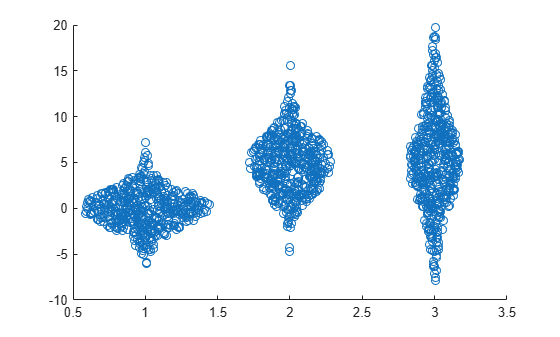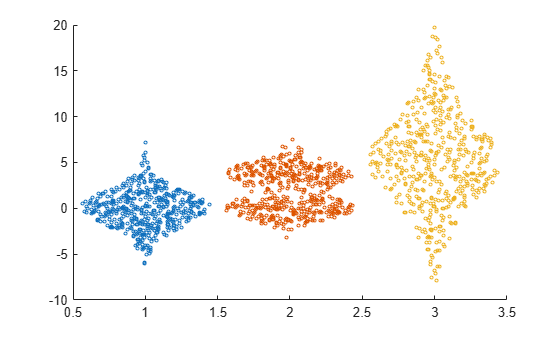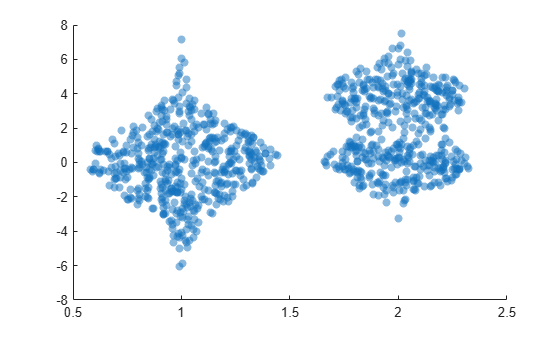# swarmchart

Swarm scatter chart

## Syntax

``swarmchart(x,y)``
``swarmchart(x,y,sz)``
``swarmchart(x,y,sz,c)``
``swarmchart(___,mkr)``
``swarmchart(___,'filled')``
``swarmchart(___,Name,Value)``
``swarmchart(ax,___)``
``s = swarmchart(___)``

## Description

example

````swarmchart(x,y)` displays a swarm chart, which is a scatter plot with the points offset (jittered) in the `x`-dimension. The points form distinct shapes, and the outline of each shape is similar to a violin plot. Swarm charts help you to visualize discrete `x` data with the distribution of the `y` data. At each location in `x`, the points are jittered based on the kernel density estimate of `y`. ```

example

````swarmchart(x,y,sz)` specifies the marker sizes. To plot all the markers with the same size, specify `sz` as a scalar. To plot the markers with different sizes, specify `sz` as a vector that is the same size as `x` and `y`.```

example

````swarmchart(x,y,sz,c)` specifies the marker colors. To plot all the markers with the same color, specify `c` as a color name or an RGB triplet. To assign a different color to each marker, specify a vector the same size as x and y. Alternatively, you can specify a three-column matrix of RGB triplets. The number of rows in the matrix must match the length of `x` and `y`.```

example

````swarmchart(___,mkr)` specifies a different marker than the default marker, which is a circle. Specify `mkr` after all the arguments in any of the previous syntaxes.```

example

````swarmchart(___,'filled')` fills in the markers. Specify the `'filled'` option after all the arguments in any of the previous syntaxes.```

example

````swarmchart(___,Name,Value)` specifies additional properties for the swarm chart using one or more `Name,Value` pair arguments. Specify the properties after all other input arguments. For a list of properties, see Scatter Properties.```

example

````swarmchart(ax,___)` displays the swarm chart in the target axes. Specify the axes before all the arguments in any of the previous syntaxes.```

example

````s = swarmchart(___)` returns the `Scatter` object. Use `s` to modify properties of the chart after creating it. For a list of properties, see Scatter Properties.```

## Examples

collapse all

Create a vector of `x` coordinates, and use the `randn` function to generate normally distributed random values for `y`. Then create a swarm chart of `x` and `y`.

```x = [ones(1,500) 2*ones(1,500) 3*ones(1,500)]; y1 = 2 * randn(1,500); y2 = 3 * randn(1,500) + 5; y3 = 5 * randn(1,500) + 5; y = [y1 y2 y3]; swarmchart(x,y)```Create three sets of `x` and `y` coordinates. Use the `randn` function to generate random values for `y`.

```x1 = ones(1,500); x2 = 2 * ones(1,500); x3 = 3 * ones(1,500); y1 = 2 * randn(1,500); y2 = [randn(1,250) randn(1,250) + 4]; y3 = 5 * randn(1,500) + 5;```

Create a swarm chart of the first data set, and specify a uniform marker size of `5`. Then call `hold on` to plot the second and third data sets together with the first data set. Call `hold off` to release the hold state of the axes.

```swarmchart(x1,y1,5) hold on swarmchart(x2,y2,5) swarmchart(x3,y3,5) hold off```Read the `BicycleCounts.csv` data set into a timetable called `tbl`. This data set contains bicycle traffic data over a period of time. Display the first five rows of `tbl`.

```tbl = readtable(fullfile(matlabroot,'examples','matlab','data','BicycleCounts.csv')); tbl(1:5,:)```
```ans=5×5 table Timestamp Day Total Westbound Eastbound ___________________ _____________ _____ _________ _________ 2015-06-24 00:00:00 {'Wednesday'} 13 9 4 2015-06-24 01:00:00 {'Wednesday'} 3 3 0 2015-06-24 02:00:00 {'Wednesday'} 1 1 0 2015-06-24 03:00:00 {'Wednesday'} 1 1 0 2015-06-24 04:00:00 {'Wednesday'} 1 1 0 ```

Create a vector `x` with the day name from each observation, and another vector y with the bicycle traffic observed. Then create a swarm chart of `x` and `y`, and specify the point marker `('.')`. The chart shows the distribution of bicycle traffic according to the day of the week.

```daynames = ["Sunday" "Monday" "Tuesday" "Wednesday" "Thursday" "Friday" "Saturday"]; x = categorical(tbl.Day,daynames); y = tbl.Total; swarmchart(x,y,'.');```Read the `BicycleCounts.csv` data set into a timetable called `tbl`. Create a vector `x` with the day name for each observation, another vector `y` with the bicycle traffic observed, and a third vector `c` with the hour of the day.

Then create a swarm chart of `x` and `y`, and specify the marker size as `20`. Specify the colors of the markers as vector `c`. The values in the vector index into the figure's colormap. Thus, the colors change according to the hour for each data point. Use the `'filled'` option to fill the markers with color instead of displaying them as hollow circles.

```tbl = readtable(fullfile(matlabroot,'examples','matlab','data','BicycleCounts.csv')); daynames = ["Sunday" "Monday" "Tuesday" "Wednesday" "Thursday" "Friday" "Saturday"]; x = categorical(tbl.Day,daynames); y = tbl.Total; c = hour(tbl.Timestamp); swarmchart(x,y,20,c,'filled');```Read the `BicycleCounts.csv` data set into a timetable called `tbl`. Create a vector `x` with the day name for each observation, another vector `y` with the bicycle traffic observed, and a third vector `c` with the hour of the day. Then create a swarm chart of `x` and `y`, and specify the marker size as `5`, and the colors of the markers as vector `c`. Call the `swarmchart` function with the return argument `s`, so that you can modify the chart after creating it.

```tbl = readtable(fullfile(matlabroot,'examples','matlab','data','BicycleCounts.csv')); daynames = ["Sunday" "Monday" "Tuesday" "Wednesday" "Thursday" "Friday" "Saturday"]; x = categorical(tbl.Day,daynames); y = tbl.Total; c = hour(tbl.Timestamp); s = swarmchart(x,y,5,c);```Change the shapes of the clusters at each `x` location, so that the points are uniformly and randomly distributed and the spacing is limited to no more than `0.5` data units.

```s.XJitter = 'rand'; s.XJitterWidth = 0.5;```Create a pair of `x` and `y` coordinates. Use the `randn` function to generate random values for `y`. Then create a swarm chart with filled markers that are 50% transparent both on their faces and on their edges.

```x1 = ones(1,500); x2 = 2 * ones(1,500); x = [x1 x2]; y1 = 2 * randn(1,500); y2 = [randn(1,250) randn(1,250) + 4]; y = [y1 y2]; swarmchart(x,y,'filled','MarkerFaceAlpha',0.5,'MarkerEdgeAlpha',0.5)```Read the `BicycleCounts.csv` data set into a timetable called `tbl`. This data set contains bicycle traffic data over a period of time. Display the first five rows of `tbl`.

```tbl = readtable(fullfile(matlabroot,'examples','matlab','data','BicycleCounts.csv')); tbl(1:5,:)```
```ans=5×5 table Timestamp Day Total Westbound Eastbound ___________________ _____________ _____ _________ _________ 2015-06-24 00:00:00 {'Wednesday'} 13 9 4 2015-06-24 01:00:00 {'Wednesday'} 3 3 0 2015-06-24 02:00:00 {'Wednesday'} 1 1 0 2015-06-24 03:00:00 {'Wednesday'} 1 1 0 2015-06-24 04:00:00 {'Wednesday'} 1 1 0 ```

Define `x` as a categorical array of the day names in the table. Define `yEast` and `yWest` as vectors containing the eastbound and westbound bicycle traffic counts.

```daynames = ["Sunday" "Monday" "Tuesday" "Wednesday" "Thursday" "Friday" "Saturday"]; x = categorical(tbl.Day,daynames); yEast = tbl.Eastbound; yWest = tbl.Westbound;```

Create a tiled chart layout in the `'flow'` tile arrangement, so that the axes fill the available space in the layout. Call the `nexttile` function to create an axes object and return it as `ax1`. Then create a swarm chart of the eastbound data by passing `ax1` to the `swarmchart` function.

```tiledlayout('flow') ax1 = nexttile; y = tbl.Eastbound; swarmchart(ax1,x,y,'.');```Repeat the process to create a second axes object and a swarm chart for the westbound traffic.

```ax2 = nexttile; y = tbl.Westbound; s = swarmchart(ax2,x,y,'.');```## Input Arguments

collapse all

x-coordinates, specified as a numeric scalar or a vector the same size as `y`.

Data Types: `single` | `double` | `int8` | `int16` | `int32` | `int64` | `uint8` | `uint16` | `uint32` | `uint64` | `categorical`

y-coordinates, specified as a numeric scalar or a vector the same size as `x`.

Data Types: `single` | `double` | `int8` | `int16` | `int32` | `int64` | `uint8` | `uint16` | `uint32` | `uint64` | `categorical` | `datetime` | `duration`

Marker size in points, specified in one of these forms:

• Numeric scalar — Plot all markers with equal size.

• Row or column vector — Use different sizes for each marker. The length of `sz` must equal the length of `x` and `y`.

• `[]` — Use the default size of 36 points.

Marker color, specified in one of these forms:

• RGB triplet or color name — Plot all the markers with the same color. An RGB triplet is a three-element row vector whose elements specify the intensities of the red, green, and blue components of the color. The intensities must be in the range `[0,1]`. Alternatively, you can specify a color name from the table below.

• Three column matrix of RGB triplets — Use different colors for each marker. Each row of the matrix specifies an RGB triplet color for the corresponding marker. The number of rows must equal the length of `x` and `y`.

• Vector — Use different colors for each marker. The values in `c` index into the current colormap, and they cover the full range of the colormap. The length of `c` must equal the length of `x` and `y`. To change the colormap, use the `colormap` function.

Color NameDescriptionEquivalent RGB Triplet
`'red'` or `'r'`Red`[1 0 0]`
`'green'` or `'g'`Green`[0 1 0]`
`'blue'` or `'b'`Blue`[0 0 1]`
`'yellow'` or `'y'`Yellow`[1 1 0]`
`'magenta'` or `'m'`Magenta`[1 0 1]`
`'cyan'` or `'c'`Cyan`[0 1 1]`
`'white'` or `'w'`White`[1 1 1]`
`'black'` or `'k'`Black`[0 0 0]`

Marker type, specified as one of the values listed in this table.

MarkerDescription
`'o'`Circle
`'+'`Plus sign
`'*'`Asterisk
`'.'`Point
`'x'`Cross
`'_'`Horizontal line
`'|'`Vertical line
`'s'`Square
`'d'`Diamond
`'^'`Upward-pointing triangle
`'v'`Downward-pointing triangle
`'>'`Right-pointing triangle
`'<'`Left-pointing triangle
`'p'`Pentagram
`'h'`Hexagram

Option to fill the interior of the markers, specified as `'filled'`. Use this option with markers that have a face, for example, `'o'` or `'square'`. Markers that do not have a face and contain only edges do not render at all (`'+'`, `'*'`, `'.'`, and `'x'`).

The `'filled'` option sets the `MarkerFaceColor` property of the `Scatter` object to `'flat'` and the `MarkerEdgeColor` property to `'none'`. In this case, MATLAB® draws the marker faces, but not the edges.

Target axes, specified as an `Axes` object, a `PolarAxes` object, or a `GeographicAxes` object. If you do not specify the axes, MATLAB plots into the current axes, or it creates an `Axes` object if one does not exist.

### Name-Value Pair Arguments

Specify optional comma-separated pairs of `Name,Value` arguments. `Name` is the argument name and `Value` is the corresponding value. `Name` must appear inside quotes. You can specify several name and value pair arguments in any order as `Name1,Value1,...,NameN,ValueN`.

Example: `swarmchart(randi(4,500,1),randn(500,1),'MarkerFaceColor','red')` specifies red filled markers.

Note

The properties listed here are only a subset. For a complete list, see Scatter Properties.

Type of jitter (spacing of points) along the x-dimension, specified as one of the following values:

• `'none'` — Do not jitter the points.

• `'density'` — Jitter the points using the kernel density estimate of y for 2-D charts. If you specify this option in two dimensions for a 3-D chart, the points are jittered based on the kernel density estimate in the third dimension. For example, setting `XJitter` and `YJitter` to `'density'` uses the kernel density estimate of z.

• `'rand'` — Jitter the points randomly with a uniform distribution.

• `'randn'` — Jitter points randomly with a normal distribution.

Maximum amount of jitter (offset between points) along the x-dimension, specified as a nonnegative scalar value in data units.

For example, to set the jitter width to 90% of the shortest distance between adjacent points, take the minimum distance between unique values of `x` and scale by `0.9`.

`XJitterWidth = 0.9 * min(diff(unique(x)));`

## Algorithms

The points in a swarm chart are jittered using uniform random values that are weighted by the Gaussian kernel density estimate of `y` and the relative number of points at each `x` location. This behavior corresponds to the default `'density'` setting of the `XJitter` property on the `Scatter` object when you call the `swarmchart` function.

The maximum spread of points at each `x` location is 90% of the smallest distance between adjacent `x` values by default:

`spread = 0.9 * min(diff(unique(x)));`

You can control the spread by setting the `XJitterWidth` property on the `Scatter` object.

### Properties

Introduced in R2020b